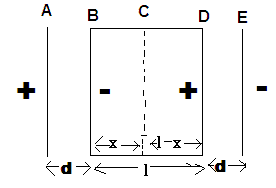# Electric field between two electrodes with insulator/conductor between.

• Ryland

#### Ryland

Hi,
I don't have a set question that needs answering, I'm just a little confused and need some clarifying if that's possible..

We've been investigating equipotentials and electric field lines between two electrodes with conductors and insulators between the electrodes.

With a conducting cylinder between the electrodes we got the equipotential lines sort of bulged around the cylinder and so the electric field lines pointed into the cylinder.
And with the insulator between we got the equipotentials curving towards the cylinder and the electric field lines curving around it.

I know that inside a conductor the electric field is zero, so does that mean on the edge is zero as well, so that's why the electric field lines hit the edge perpendicular?

but i don't really know why that happens with the insulator, is it just because electric field cannot go through an insulator so it 'bends' around the cylinder?

Any help or direction you can give me will be greatly appreciated.
Thanks.

hi i am also stuck with the same problem.I WILL EXPLAIN MY LOGIC.SO PLEASE BEAR WITH MELet a charge 1C be given to plate A and equal -1C given to E.Now due to induction B will attain some -ve charge and D with an equal positive charge.Charges flow from D to B till magnitudes of charges on A,B,D,E become same
so now we have A=1C,B=-1C,D=1C,E=-1C
now let us assume the net electric field intensity is 0 somewere at C
so AC=(d+x),BC=x,CD=(l-x),CD=(d+l-x)

now field due to A on C=(K*1)/(d+x)^2 from A to C=[A]
B on C=(K*1)/(x)^2 from C to B=
D on C=(K*1)/(l-x)^2 from D to C=[D]
E on C=(K*1)/(d+l-x)^2 from C to E=[E]

therefore for electric field to be 0 at C

B+D-(A+E)=0
putting values

(k)/(x)^2+(K)/(l-x)^2=(K)/(d+x)^2+(K)/(d+l-x)^2

on solving for x you can obtain at what point field is 0. thank u

i have gave by reasoning.i don't think this should be wrong.but please if u can verify it will be good

Hi,
With a conducting cylinder between the electrodes we got the equipotential lines sort of bulged around the cylinder and so the electric field lines pointed into the cylinder.
And with the insulator between we got the equipotentials curving towards the cylinder and the electric field lines curving around it.

First of all, what exactly did you do to investigate the fields lines?

The field inside a conductor is zero. At the surface, the field is perpendicular (to the surface). The external field induces charges on the conductor, the field inside is zero and is perpendicular at the surface. The charges induced in the conductor produce their own field outside the conductor, which would slightly cancel the field outside, the field outside the conductor would increase from zero in conductor to its original value far from the conductor (or here, at the two electrodes. Also, the field need not be zero everywhere on the surface of the conductor). So you would have equipotential lines bulged around the conductor.

In case of the insulator, the field would just polarize the atoms. The field would be reduced inside the insulator, but not zero, & it would still be pointing in the same direction. There would be some surface charges due to the polarization, their field again very slightly cancels the field outside, leading to the equipotentials bending towards the cylinder.